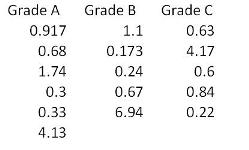# IASSC Lean Six Sigma – Black Belt v6.1

Page:    1 / 20
Exam contains 304 questions

When we gather information for the Voice of the Business we are primarily interested in information concerning the _____________ of the business.

• B. Market share
• C. Profitability
• D. Ownership

The relationship between a response variable and one or more independent variables is investigated and modeled by use of __________________.

• A. X-Y Matrix
• B. Baldridge Assessment
• C. Analysis of Variance (ANOVA)
• D. Critical X's Definition

When doing Hypothesis Testing on Non-normal data Belts will use a ________________ to compare more than two sample proportions to each other.

• A. Z score Table
• B. Sakami Table
• C. Mean-to-Mode Analysis
• D. Contingency Table

The distance between the Mean of a data set and the Point of Inflection on a Normal curve is called the _______________.

• B. Standard Deviation
• C. Numerical Average

Which statement(s) are incorrect about Fractional Factorial Designs?

• A. A Half Fractional Design for 5 factors has the same number of experimental runs as a Full Factorial Design for 4 factors assuming no repeats or replicates or Center Points
• B. Quarter Fractional experiments can exist for those with 4 factors
• C. Resolution V design is desired while controlling costs of experimentation
• D. Half Fractional experiments do not exist for those designs with only 2 factors

As a means of measuring the effects on other areas of a process as a result of changes in the primary metric we also define and track ________________.

• A. Parallel process metrics
• B. Secondary metrics
• C. Tertiary metrics
• D. Industry standards

Kanban establishes a means of monitoring production, conveyance and delivery information such that efficient flow is established. The method used by Kanban is to require a _________ before anything moves.

• A. Sign-off
• B. Signal
• C. Bell to ring
• D. Work order

For the data set shown here which of these statements is/are true?• A. Hypothesis Testing of Means or Medians cannot be done since there are an unequal number of observations for the 3 samples
• B. A Paired T-test would be applicable for comparing Grade B and Grade A since they follow each other in the data set
• C. Grade A has the lowest sample Mean of the 3 samples
• D. Grade A has a higher sample Mean than Grade B

One of the foundations of Lean Six Sigma is the concept that the output of a process (Y) is influenced by the process inputs (Xs) and is commonly shown as which formula?

• A. Y = Z(X2)
• B. Y = f(X3)
• C. Y = f(Xn)
• D. Y = g(X+ 1.5)

One of the primary deliverables from performing a SIPOC is to begin to understand which inputs have the greatest affect on the ______________ outputs.

• A. Management’s desired
• B. Supplier delivered
• C. Process operator
• D. Customer most valued

A valid Multiple Linear Regression (MLR) is characterized by all of these except
_______________________.

• A. It is an assumption that the X’s (inputs) are not correlated to each other
• B. The X’s (inputs) are assumed to be independent of each other
• C. MLR is conducted based on a deliberate form of experimentation
• D. The Residuals from MLR analysis have to be Normally Distributed

When one speaks of 20% of something contributing 80% of the affect they are referring to what is known as the _________________.

• A. Shewhart Example
• B. Connection Principle
• C. Balance Equation
• D. Pareto Principle

All the data points that represent the total set of information of interest is called the
________________ .

• A. Population
• B. Sample
• C. Frame

A Belt working in a supply chain environment has to make a decision to change suppliers of critical raw materials for a new product upgrade. The purchasing manager is depending on the Belts effort requiring that the average cost of an internal critical raw material component be less than or equal to \$3,800 in order to stay within budget. Using a sample of 38 first article components, a Mean of the new product upgrade price of \$3,680, and a
Standard Deviation of \$120 was estimated. In order to increase the Long Term Z value to
5, what is the maximum long term variation in pricing the Belt can accept for his upgraded critical raw material component?

• A. \$6
• B. \$12
• C. \$24
• D. \$48

The most appropriate type of FMEA for a product before going into manufacturing is a

• A. Design
• B. Consumer
• C. Survey
• D. Test Process